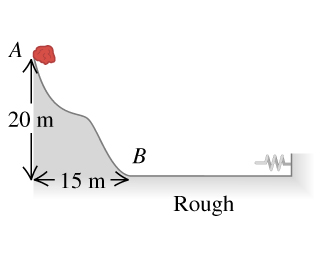# Problem: A 13.0 kg stone slides down a snow-covered hill (the figure ), leaving point exttip{A}{A} with a speed of 12.0 m/s . There is no friction on the hill between points exttip{A}{A} and exttip{B}{B}, but there is friction on the level ground at the bottom of the hill, between exttip{B}{B} and the wall. After entering the rough horizontal region, the stone travels 100 m and then runs into a very long, light spring with force constant 2.30 N/m . The coefficients of kinetic and static friction between the stone and the horizontal ground are 0.20 and 0.80, respectively.What is the speed of the stone when it reaches point exttip{B}{B}?How far will the stone compress the spring?Will the stone move again after it has been stopped by the spring?

⚠️Our tutors found the solution shown to be helpful for the problem you're searching for. We don't have the exact solution yet.

###### Problem Details

A 13.0 kg stone slides down a snow-covered hill (the figure), leaving point with a speed of 12.0 m/s . There is no friction on the hill between points and , but there is friction on the level ground at the bottom of the hill, between and the wall. After entering the rough horizontal region, the stone travels 100 m and then runs into a very long, light spring with force constant 2.30 N/m . The coefficients of kinetic and static friction between the stone and the horizontal ground are 0.20 and 0.80, respectively.

What is the speed of the stone when it reaches point ?

How far will the stone compress the spring?

Will the stone move again after it has been stopped by the spring?